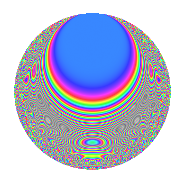# Properties

 Label 4031.2.a.cLevel 4031 Weight 2 Character orbit 4031.a Self dual Yes Analytic conductor 32.188 Analytic rank 1 Dimension 61 CM No

# Learn more about

## Newspace parameters

 Level: $$N$$ = $$4031 = 29 \cdot 139$$ Weight: $$k$$ = $$2$$ Character orbit: $$[\chi]$$ = 4031.a (trivial)

## Newform invariants

 Self dual: Yes Analytic conductor: $$32.1876970548$$ Analytic rank: $$1$$ Dimension: $$61$$ Fricke sign: $$1$$ Sato-Tate group: $\mathrm{SU}(2)$

## $q$-expansion

The dimension is sufficiently large that we do not compute an algebraic $$q$$-expansion, but we have computed the trace expansion.

 $$\operatorname{Tr}(f)(q) =$$ $$61q$$ $$\mathstrut -\mathstrut q^{2}$$ $$\mathstrut -\mathstrut 4q^{3}$$ $$\mathstrut +\mathstrut 43q^{4}$$ $$\mathstrut -\mathstrut 7q^{5}$$ $$\mathstrut -\mathstrut 13q^{6}$$ $$\mathstrut -\mathstrut 10q^{7}$$ $$\mathstrut -\mathstrut 6q^{8}$$ $$\mathstrut +\mathstrut 23q^{9}$$ $$\mathstrut +\mathstrut O(q^{10})$$ $$\operatorname{Tr}(f)(q) =$$ $$61q$$ $$\mathstrut -\mathstrut q^{2}$$ $$\mathstrut -\mathstrut 4q^{3}$$ $$\mathstrut +\mathstrut 43q^{4}$$ $$\mathstrut -\mathstrut 7q^{5}$$ $$\mathstrut -\mathstrut 13q^{6}$$ $$\mathstrut -\mathstrut 10q^{7}$$ $$\mathstrut -\mathstrut 6q^{8}$$ $$\mathstrut +\mathstrut 23q^{9}$$ $$\mathstrut -\mathstrut 16q^{10}$$ $$\mathstrut -\mathstrut 3q^{11}$$ $$\mathstrut -\mathstrut 18q^{12}$$ $$\mathstrut -\mathstrut 28q^{13}$$ $$\mathstrut -\mathstrut 14q^{14}$$ $$\mathstrut -\mathstrut 12q^{15}$$ $$\mathstrut +\mathstrut 11q^{16}$$ $$\mathstrut -\mathstrut 21q^{17}$$ $$\mathstrut -\mathstrut 17q^{18}$$ $$\mathstrut -\mathstrut 36q^{19}$$ $$\mathstrut -\mathstrut 16q^{20}$$ $$\mathstrut -\mathstrut 12q^{21}$$ $$\mathstrut -\mathstrut 42q^{22}$$ $$\mathstrut -\mathstrut 15q^{23}$$ $$\mathstrut -\mathstrut 28q^{24}$$ $$\mathstrut -\mathstrut 16q^{25}$$ $$\mathstrut -\mathstrut 13q^{26}$$ $$\mathstrut -\mathstrut 10q^{27}$$ $$\mathstrut -\mathstrut 25q^{28}$$ $$\mathstrut -\mathstrut 61q^{29}$$ $$\mathstrut -\mathstrut 12q^{30}$$ $$\mathstrut -\mathstrut 18q^{31}$$ $$\mathstrut -\mathstrut 3q^{32}$$ $$\mathstrut -\mathstrut 42q^{33}$$ $$\mathstrut -\mathstrut 22q^{34}$$ $$\mathstrut -\mathstrut 29q^{35}$$ $$\mathstrut -\mathstrut 38q^{36}$$ $$\mathstrut -\mathstrut 30q^{37}$$ $$\mathstrut -\mathstrut 27q^{38}$$ $$\mathstrut -\mathstrut 31q^{39}$$ $$\mathstrut -\mathstrut 22q^{40}$$ $$\mathstrut -\mathstrut 28q^{41}$$ $$\mathstrut -\mathstrut 9q^{42}$$ $$\mathstrut -\mathstrut 58q^{43}$$ $$\mathstrut -\mathstrut 2q^{44}$$ $$\mathstrut -\mathstrut 31q^{45}$$ $$\mathstrut -\mathstrut 40q^{46}$$ $$\mathstrut -\mathstrut 6q^{47}$$ $$\mathstrut -\mathstrut 37q^{48}$$ $$\mathstrut -\mathstrut 37q^{49}$$ $$\mathstrut -\mathstrut 15q^{50}$$ $$\mathstrut -\mathstrut 44q^{51}$$ $$\mathstrut -\mathstrut 43q^{52}$$ $$\mathstrut -\mathstrut 27q^{53}$$ $$\mathstrut -\mathstrut 18q^{54}$$ $$\mathstrut -\mathstrut 38q^{55}$$ $$\mathstrut -\mathstrut 22q^{56}$$ $$\mathstrut -\mathstrut 50q^{57}$$ $$\mathstrut +\mathstrut q^{58}$$ $$\mathstrut -\mathstrut 24q^{59}$$ $$\mathstrut +\mathstrut 6q^{60}$$ $$\mathstrut -\mathstrut 76q^{61}$$ $$\mathstrut -\mathstrut 17q^{62}$$ $$\mathstrut -\mathstrut 6q^{63}$$ $$\mathstrut -\mathstrut 60q^{64}$$ $$\mathstrut -\mathstrut 65q^{65}$$ $$\mathstrut -\mathstrut 7q^{66}$$ $$\mathstrut -\mathstrut 45q^{67}$$ $$\mathstrut -\mathstrut 31q^{68}$$ $$\mathstrut -\mathstrut 16q^{69}$$ $$\mathstrut -\mathstrut 48q^{70}$$ $$\mathstrut -\mathstrut 28q^{71}$$ $$\mathstrut -\mathstrut 40q^{72}$$ $$\mathstrut -\mathstrut 50q^{73}$$ $$\mathstrut -\mathstrut 17q^{74}$$ $$\mathstrut -\mathstrut 35q^{75}$$ $$\mathstrut -\mathstrut 100q^{76}$$ $$\mathstrut +\mathstrut q^{77}$$ $$\mathstrut -\mathstrut 6q^{78}$$ $$\mathstrut -\mathstrut 66q^{79}$$ $$\mathstrut -\mathstrut 10q^{80}$$ $$\mathstrut -\mathstrut 63q^{81}$$ $$\mathstrut -\mathstrut 5q^{82}$$ $$\mathstrut -\mathstrut 9q^{83}$$ $$\mathstrut -\mathstrut 24q^{84}$$ $$\mathstrut -\mathstrut 77q^{85}$$ $$\mathstrut +\mathstrut 29q^{86}$$ $$\mathstrut +\mathstrut 4q^{87}$$ $$\mathstrut -\mathstrut 62q^{88}$$ $$\mathstrut -\mathstrut 30q^{89}$$ $$\mathstrut +\mathstrut 50q^{90}$$ $$\mathstrut -\mathstrut 52q^{91}$$ $$\mathstrut -\mathstrut 53q^{92}$$ $$\mathstrut -\mathstrut 42q^{93}$$ $$\mathstrut -\mathstrut 92q^{94}$$ $$\mathstrut -\mathstrut 20q^{95}$$ $$\mathstrut -\mathstrut 47q^{96}$$ $$\mathstrut -\mathstrut 34q^{97}$$ $$\mathstrut +\mathstrut 36q^{98}$$ $$\mathstrut -\mathstrut 29q^{99}$$ $$\mathstrut +\mathstrut O(q^{100})$$

## Embeddings

For each embedding $$\iota_m$$ of the coefficient field, the values $$\iota_m(a_n)$$ are shown below.

For more information on an embedded modular form you can click on its label.

Label $$a_{2}$$ $$a_{3}$$ $$a_{4}$$ $$a_{5}$$ $$a_{6}$$ $$a_{7}$$ $$a_{8}$$ $$a_{9}$$ $$a_{10}$$
1.1 −2.62831 0.935172 4.90799 −2.36331 −2.45792 0.343928 −7.64309 −2.12545 6.21151
1.2 −2.62306 1.48834 4.88043 0.915377 −3.90400 −2.34390 −7.55555 −0.784847 −2.40109
1.3 −2.53428 −2.04027 4.42257 −2.47983 5.17061 −0.629929 −6.13948 1.16269 6.28458
1.4 −2.49843 −0.867725 4.24214 3.27534 2.16795 1.98132 −5.60183 −2.24705 −8.18321
1.5 −2.42000 −2.29529 3.85639 1.32254 5.55461 0.993943 −4.49245 2.26837 −3.20054
1.6 −2.31412 0.311751 3.35513 1.29585 −0.721429 −1.13716 −3.13593 −2.90281 −2.99874
1.7 −2.25610 3.35950 3.09001 −1.55616 −7.57937 −0.413231 −2.45917 8.28621 3.51085
1.8 −2.23009 1.62667 2.97329 2.06861 −3.62762 3.32810 −2.17052 −0.353941 −4.61317
1.9 −2.10281 −1.04272 2.42182 3.50696 2.19264 −3.91864 −0.887016 −1.91274 −7.37448
1.10 −1.99079 −3.03517 1.96324 −1.96489 6.04239 0.618316 0.0731745 6.21228 3.91168
1.11 −1.87723 1.73503 1.52398 −2.87174 −3.25705 −0.555991 0.893598 0.0103383 5.39090
1.12 −1.83395 2.22148 1.36337 1.24306 −4.07408 2.01007 1.16755 1.93497 −2.27970
1.13 −1.77273 −1.39503 1.14258 −2.16266 2.47302 3.73562 1.51997 −1.05388 3.83382
1.14 −1.73344 −1.78807 1.00480 −2.76512 3.09951 −4.11682 1.72512 0.197207 4.79315
1.15 −1.55145 0.350675 0.406987 −2.80587 −0.544053 −3.32540 2.47147 −2.87703 4.35316
1.16 −1.50584 −0.996538 0.267567 0.533194 1.50063 3.56628 2.60877 −2.00691 −0.802908
1.17 −1.44140 2.16928 0.0776255 0.0237256 −3.12680 1.29161 2.77090 1.70580 −0.0341981
1.18 −1.44025 1.49462 0.0743258 2.03878 −2.15264 −3.27699 2.77346 −0.766098 −2.93636
1.19 −1.19064 −0.642478 −0.582384 −0.355693 0.764958 0.205071 3.07468 −2.58722 0.423501
1.20 −1.13471 1.15618 −0.712442 3.10962 −1.31192 −1.02298 3.07782 −1.66325 −3.52850
See all 61 embeddings
 $$n$$: e.g. 2-40 or 990-1000 Embeddings: e.g. 1-3 or 1.61 Significant digits: Format: Complex embeddings Normalized embeddings Satake parameters Satake angles

## Inner twists

This newform does not have CM; other inner twists have not been computed.

## Atkin-Lehner signs

$$p$$ Sign
$$29$$ $$1$$
$$139$$ $$1$$

## Hecke kernels

This newform can be constructed as the kernel of the linear operator $$T_{2}^{61} + \cdots$$ acting on $$S_{2}^{\mathrm{new}}(\Gamma_0(4031))$$.